# 1751 A Pythagorean Triple Puzzle

### Today’s Puzzle:

Even though they don’t look like it, the fourteen triangles in today’s puzzle are all Pythagorean triple triangles that most certainly have not been drawn to scale. The marked angle is the right angle for each of triangle. The hypotenuse of each triangle is less than 100. There is a chart under the puzzle listing all possible Pythagorean triples that could be used in the puzzle. No triple will be used more than once. Use logic to figure out what the missing side lengths are. Have fun!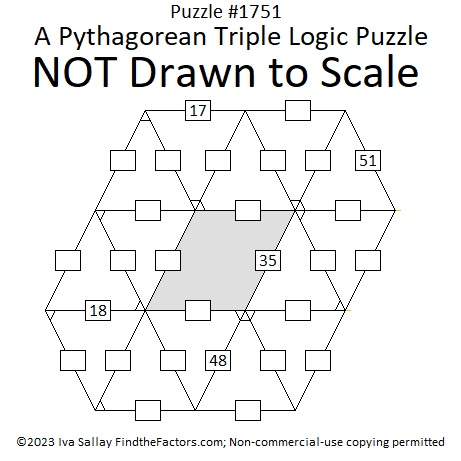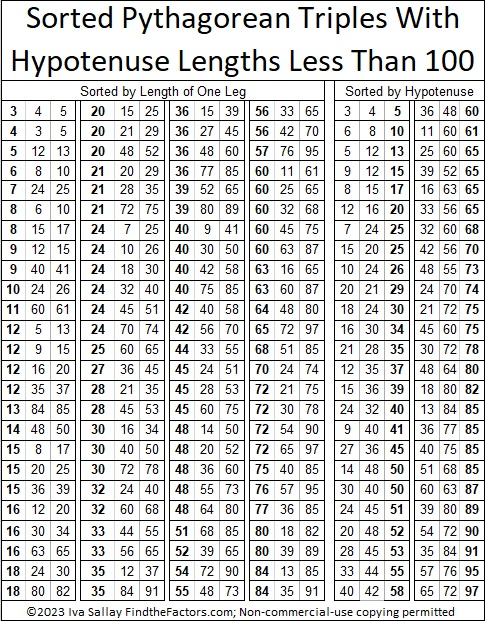### Factors of 1751:

If you remember that 51 is NOT a prime number because 3 × 17 = 51, then it isn’t difficult to recognize that 1751 also must be divisible by 17.

• 1751 is a composite number.
• Prime factorization: 1751 = 17 × 103.
• 1751 has no exponents greater than 1 in its prime factorization, so √1751 cannot be simplified.
• The exponents in the prime factorization are 1 and 1. Adding one to each exponent and multiplying we get (1 + 1)(1 + 1) = 2 × 2 = 4. Therefore 1751 has exactly 4 factors.
• The factors of 1751 are outlined with their factor pair partners in the graphic below.### More About the Number 1751:

1751 is the hypotenuse of a Pythagorean triple:
824-1545-1751 which is (8-15-17) times 103.

1751 looks interesting in some other bases:
It’s 575 in base 18 because 5(18²) + 7(18) + 5(1) = 1751,
373 base 23 because 3(23²) + 7(23) + 3(1) = 1751, and
1F1 base 35 because 1(35²) + 15(35) + 1(1) = 1751.

# 1672 A Pythagorean Triple Puzzle with Only Two Clues

### Today’s Puzzle:

If you would like to try a Pythagorean Triple Puzzle that isn’t too difficult, I’ve got you covered with today’s puzzle:

PUZZLE DIRECTIONS: This puzzle is NOT drawn to scale. Although all of the angles may look like 60-degree angles, none of them are. The marked angles are 90 degrees. Lines that look parallel are NOT parallel. Although side lengths look equal, they are NOT equal. Most rules of geometry do not apply here. When drawn to scale, none of the triangles in this particular puzzle overlap, but that is not always the case for some of my other Pythagorean triple puzzles.

No geometry is needed to solve this puzzle. All that is needed is logic and the table of Pythagorean triples under the puzzle. The puzzle only uses triples in which each leg and each hypotenuse is less than 100 units long. The puzzle has only one solution.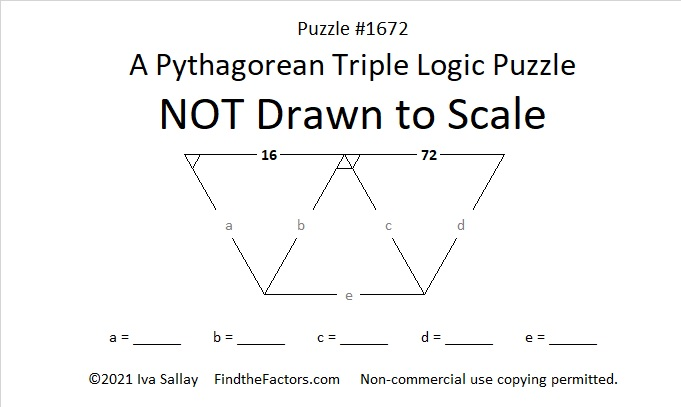You can also print and cut out the triangle cards below to solve the puzzle.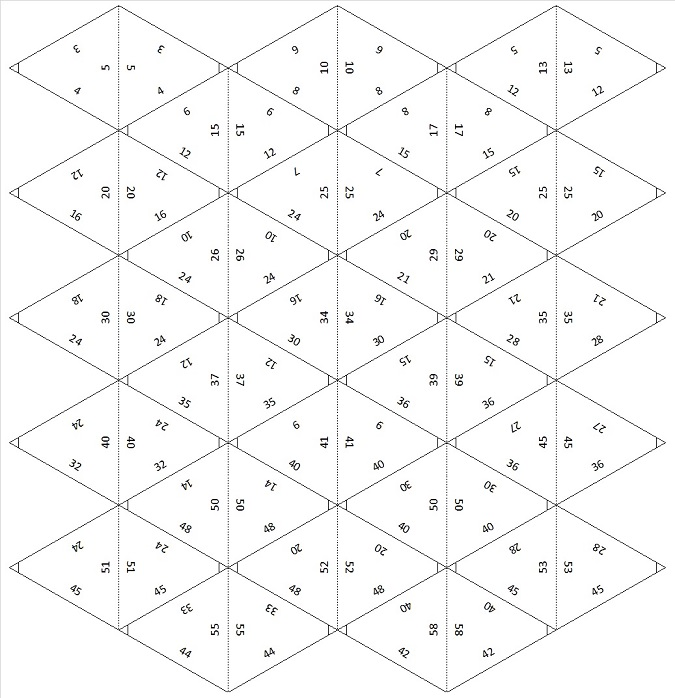### Factors of 1672:

• Prime factorization: 1672 = 2 × 2 × 2 × 11 × 19, which can be written 1672 = 2³ × 11 × 19.
• 1672 has at least one exponent greater than 1 in its prime factorization so √1672 can be simplified. Taking the factor pair from the factor pair table below with the largest square number factor, we get √1672 = (√4)(√418) = 2√418.
• The exponents in the prime factorization are 3,1 and 1. Adding one to each exponent and multiplying we get (3 + 1)(1 + 1) (1 + 1) = 4 × 2 × 2 = 16. Therefore 1672 has exactly 16 factors.
• The factors of 1672 are outlined with their factor pair partners in the graphic below.### More About the Number 1672:

1672 is the difference of two squares in FOUR different ways:
419² – 417² = 1672,
211² – 207² = 1672,
49² – 27² = 1672, and
41² – 3² = 1672.
That last one means we are only 9 numbers away from the next perfect square, 1681.

From NumbersaPlenty, we learn that 1672 is a digitally powerful number:
1672 = 1¹ + 6⁴ + 7³ + 2⁵.

# 1648 A Pythagorean Triple Logic Puzzle with a Triangular Card Deck

### Today’s Puzzle:

It’s been a few years since I’ve made one of these Pythagorean triple logic puzzles. The triangles in it are shaped a little different than in years past because I also wanted to make a deck of Pythagorean triple cards that are shaped like equilateral triangles or at least as close as I can get to equal sides. This puzzle won’t be easy, but do give it a try!

PUZZLE DIRECTIONS: This puzzle is NOT drawn to scale. Although all of the angles may look like 60-degree angles, none of them are. The marked angles are 90 degrees. Lines that look parallel are NOT parallel. Although side lengths look equal, they are NOT equal. Most rules of geometry do not apply here: in fact, non-adjacent triangles in the drawing might actually overlap.

No geometry is needed to solve this puzzle. All that is needed is logic and the table of Pythagorean triples under the puzzle. The puzzle only uses triples in which each leg and each hypotenuse is less than 100 units long. The puzzle has only one solution.Print the puzzles or type the solution in this excel file: 12 Factors 1639-1648

### Triangular Card Deck of Pythagorean Triples:

You can make this deck of 50 playing cards to help you solve the puzzle or perhaps to play a domino-type game. Print each group of 25 cards on a separate sheet of paper. Cut the cards out along the solid lines and fold the cards on the dotted lines. Use a glue stick to keep the front of each card attached to its back. Laminate the cards, if desired. The right angles are the only angles marked on the cards, and the hypotenuses are all along the folded edges. Note: Some sides will not match with any other side in the deck, and the 57-76-95 triangle does not match sides with ANY triangle with a hypotenuse less than 100.### Factors of the Number 1648:

• 1648 is a composite number.
• Prime factorization: 1648 = 2 × 2 × 2 × 2 × 103, which can be written 1648 = 2⁴ × 103.
• 1648 has at least one exponent greater than 1 in its prime factorization so √1648 can be simplified. Taking the factor pair from the factor pair table below with the largest square number factor, we get √1648 = (√16)(√103) = 4√103.
• The exponents in the prime factorization are 4 and 1. Adding one to each exponent and multiplying we get (4 + 1)(1 + 1) = 5 × 2 = 10. Therefore 1648 has exactly 10 factors.
• The factors of 1648 are outlined with their factor pair partners in the graphic below.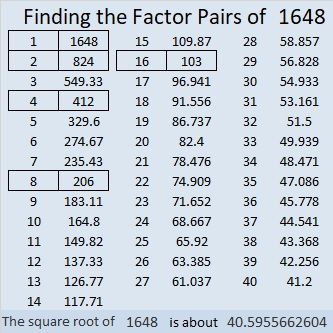### More About the Number 1648:

1648 is not the hypotenuse of any Pythagorean triples because none of its prime factors leave a remainder of 1 when divided by 4.

1648 is the difference of two squares three different ways:
413² – 411² = 1648,
208² – 204² = 1648, and
107² – 99² = 1648.

That means 1648 is a leg in THREE Pythagorean triples calculated from
413² – 411², 2(413)(411), 413² + 411²;
208² – 204², 2(208)(204), 208² + 204²; and
107² – 99², 2(107)(99), 107² + 99².

1648 can be expressed as 2(824)(1), 2(412)(2), 2(206)(4), as well as 2(103)(8).

That means 1648 is a leg in FOUR Pythagorean triples calculated from
2(824)(1), 824² – 1², 824² + 1²;
2(412)(2), 412² – 2², 412² + 2²;
2(206)(4), 206² – 4², 206² + 4²; and
2(103)(8), 103² – 8², 103² + 8².

Sometimes those formulas produce duplicate triples, but not this time. 1648 is in SEVEN Pythagorean triples!
1648-339486-339490,
1648-84864-84880,
1648-21186-21250,
1648-678975-678977,
1648-169740-169748,
1648-42420-42452,
1648-10545-10673.

Aren’t you glad that today’s puzzle was limited to triples with hypotenuses less than 100? I certainly wouldn’t want any of those triples with 1648 as a side to be part of any puzzle! But 2-digit sides, 16 and 48, helped to make today’s puzzle, and I do hope you were able to solve it today!